# Precalculus : Use the laws of cosines and sines

## Example Questions

1 2 4 Next →

### Example Question #31 : Law Of Cosines And Sines

Find the length of the missing side,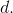Possible Answers: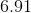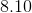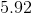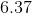Correct answer:Explanation:

First, use the Law of Sines to find the measurement of angle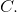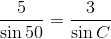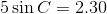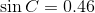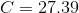Recall that all the angles in a triangle need to add up todegrees.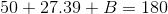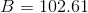Now, use the Law of Sines again to find the length of.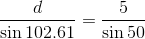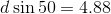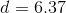### Example Question #32 : Law Of Cosines And Sines

What is the largest possible angle, measured in degrees, in triangle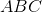if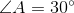,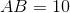, and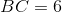?

Possible Answers: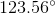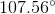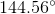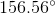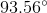Correct answer:Explanation:

In the ambiguous SSA case, use Law of Sines to solve for the angle opposite the given side.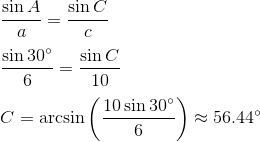If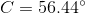, then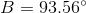However! Another possible value of C is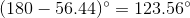In this case the angles will be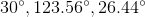.

This is bigger thanand is consequently the answer.

1 2 4 Next →

### All Precalculus Resources